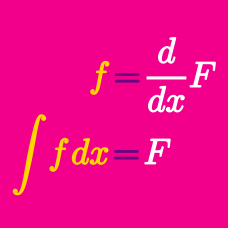Calculus

# Integration of Logarithmic Functions

If $xf'(x)=24\ln x,$ what is the function $f(x)?$ (Use $C$ as the constant of integration.)

If $f(x)$ is a function such that $f(x)=\int \frac{1}{x \sqrt{\ln x+3}} dx$ and $f(e)=21,$ what is the value of $f(e^{5})?$

What is the indefinite integral$\int \frac{1}{x}\left(7(\ln x)^{6}+5\right) dx?$ (Use $C$ as the constant of integration.)

Let $N = \displaystyle \int_{1}^{e^ 8} \ln \left( \dfrac {1}{x} \right) \, dx$. If $N = -ae^b - c$, where $a$, $b$ and $c$ are positive integers and $e$ is the base of the natural logarithm, what is the value of $a + b + c$?

If $f(x)$ is a function satisfying $f(x)=\int \frac{6(\ln x)^{5}}{x} dx$ and $f(e)=8,$ what is the value of $f(e^2)?$

×